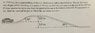# Will the car make it to the top of the hill?

• Dillion

## Homework Statement

A 1500 kg car is approaching an icy (i.e. frictionless) hill as shown below. The car then runs out of gas traveling at a speed of 10 m/s. a) Will the car make it to the top of the hill?

picture is attached

## Homework Equations

vf^2 = vi^2 + 2ax[/B]

## The Attempt at a Solution

Vf is 0. Vi is 10. x is 10 (sin30 = 5/x)

0 = 0 +10a

i'm lost here at acceleration. If the car is running out of gas, how are we supposed to know how much it is slowing down by? :(

#### Attachments

•13036372_10206439007509133_1292263014_o.jpg
31.4 KB · Views: 352
What force causes the car to slow down?

Are you familiar with kinetic energy? and gravitational potential energy?

What force causes the car to slow down?

Are you familiar with kinetic energy? and gravitational potential energy?

1/2mvi^2 + mgh = 1/2mvf^2 + mgh

75000+73500=750vf^2+147000

vf = 1.4

as for what forces cause the car to slow down...i would say friction but this problem says frictionless.

You've found the correct speed.
You're right that there is no frictional force. What other forces are acting on the car? Can you draw a free body diagram? The relevant force is the same force that's stopping you from floating up out of your chair right now..

You've found the correct speed.
You're right that there is no frictional force. What other forces are acting on the car? Can you draw a free body diagram? The relevant force is the same force that's stopping you from floating up out of your chair right now..

Gravitational force. The gravitational force will point straight down which will be (9.8 x 1500) = 14700 N.

Gravitational force. The gravitational force will point straight down which will be (9.8 x 1500) = 14700 N.
Correct. If we draw a free body diagram and define our positive x direction to be parallel to the incline and to the right then there will be some component of the gravitational force acting in the negative x direction. That is the force decelerating the car.
You could've also found the same answer you found via conservation of energy by applying Newtons second law. Though, in this case, the method you did use is faster/simpler.